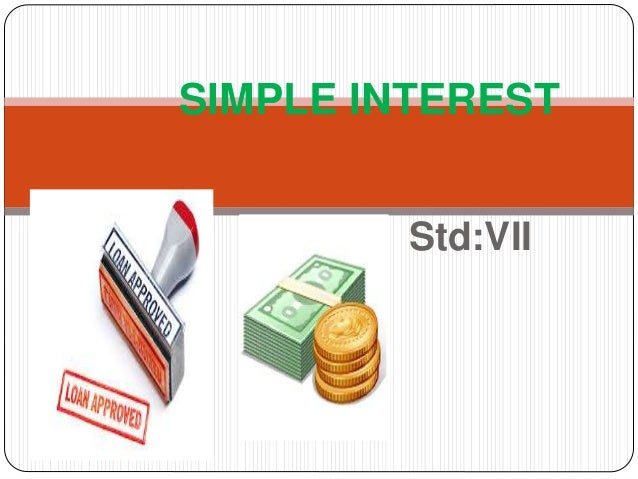Successfully reported this slideshow.
We use your LinkedIn profile and activity data to personalize ads and to show you more relevant ads. You can change your ad preferences anytime.Upcoming SlideShare
Loading in …5
×

# Simple interest

The power point explains the concept of simple interest and the development of formula of simple interest.It also attempts to solve few problems.

• Full Name
Comment goes here.

Are you sure you want to Yes No
Your message goes here• Login to see the comments

### Simple interest

1. 1. SIMPLE INTEREST Std:VII
2. 2.  What do you observe? What comes in your mind when you see this picture?  What do we call it in mathematical terms?
3. 3. imple Interest
4. 4. What is simple interest? We borrow some amount or money from a bank or a money lender This borrowed amount is called as loan. However, at the time of returning the loan to the bank or the money lender we have to pay back an amount somewhat bigger than the loan. This additional amount is called as SIMPLE INTEREST.
5. 5. T = N
6. 6. 1) You want to buy this tractor, It costs Rs.15,000. You decide to get a five year loan with a 6.5% interest rate for Rs.15,000. How much interest will you pay?
7. 7. Given Interest Paid=? Principal(loan amount) = Rs.15,000 Rate = 6.5p.c.p.a. Period(N) = 5 years Solution: I = P x N x R 100
8. 8. I = 15000x5x6.5 100 = 4,87,500 100 =4,875 To buy this tractor we will pay Interest of Rs 4,875
9. 9.  The simple interest on Rs. 45,000 in 3 years is Rs. 13,500. What is the rate of Interest? Given o Interest Paid=Rs.13,500  Principal(loan amount) = Rs.45,000  Rate = ?  Period(N) = 3years
10. 10.  Solution: I = P x N x R 100 13,500 =45000x3xR 100 R = 13500x100 45000x3 =10 p.c.p.a. The Rate of Interest = 10 p.c.p.a.
11. 11. A certain sum of money earns an interest of Rs.3,630 in 3 years at the rate of 11 p.c.p.a, what is the principal? Given o Interest Paid=Rs.3,630  Principle(loan amount) = ?  Rate = 11p.c.p.a.  Period(N) = 3years
12. 12.  Solution: I = P x N x R 100 3630 =Px3x11 100 P= 3630x100 11x3 =Rs.11,000 The Principal amount is Rs.11,000
13. 13.  The additional amount paid on a loan amount is called Simple Interest.  The Formula for Simple Interest (S.I.) is: S.I.= P x R x N 100
14. 14.  Define Simple Interest.  What Is: P = ? R= ? Solve the Following Questions: Sr. No. Principal Rs. Rate of Interest p.c.p.a. Number of years 1 9000 10 3 2 15000 11 2